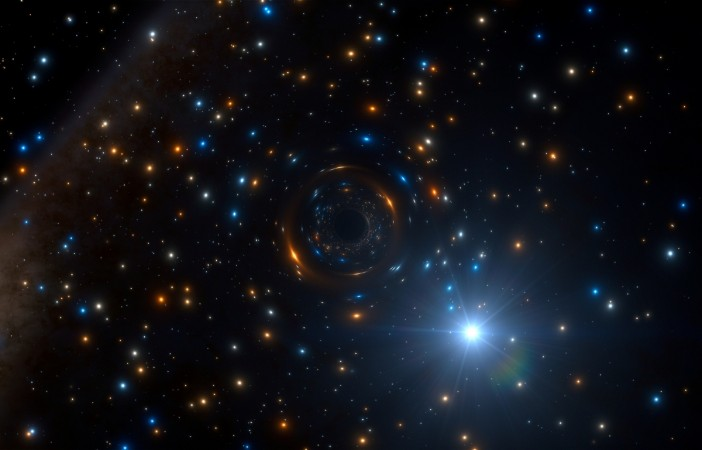# In The Universe, There Exists A Type Of Black Hole That Erases The Past And Gives Infinite Potential FuturesSHARE

According to a mathematician at the University of California Berkeley, in the Universe, there exists a type of a black hole that erases the past and gives infinite potential futures.

In the reality we all live in, the past of an individual is uniquely determining the future. Thus, if a physicist can find out where did the Universe begin, he would be able to calculate mathematically the Universe’s future, for all its possible times and potential spaces.

However, there is a type of black holes that break the physics laws, as we know them, according to the recent study. Therefore, if someone will go through such a black hole, then he or she would have to live with an infinity of potential futures.

According to UC Berkeley postdoctoral mathematician Peter Hintz, through mathematics it can be observed that in a fast-expanding Universe like the one we live in, the existence of a non-deterministic world it is possible.

The recent theory could break down a 40-year-lasting principle

The deterministic principle, proposed for the first time 40 years ago by the physicist Roger Penrose, holds sacredly on the key idea of determinism for any physical theory. That is, given the past and the present, the physical laws of the universe do not allow more than one possible future.

“No physicist is going to travel inside a black hole and measure it. It is a mathematical question. It is a question that really can only be studied mathematically, but that has physical, almost philosophical implications, which makes it very interesting,” explained Hintz.

The ideas of multiple universes, infinite futures, and so on are somehow hard to swallow by many traditional physicists but recent discoveries lead towards that. As Peter Hintz theorized that there exists a type of black hole that erases the past and gives infinite potential futures, there are many other scientists that have shown that the laws of physics, as we know them, may be different in various parts of the Universe.

SHARE